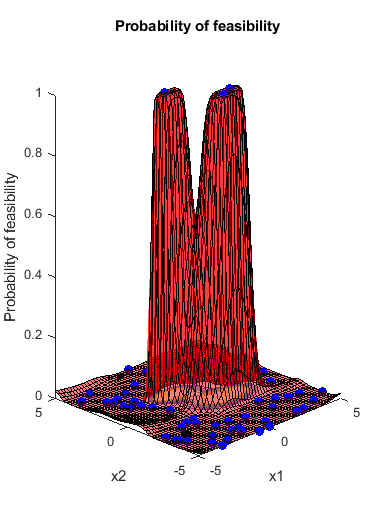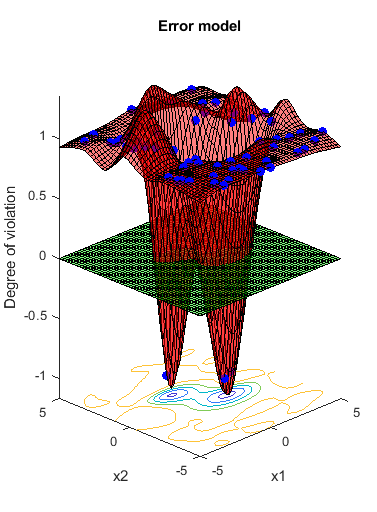Documentation

# predictError

Predict error value at a set of points

## Syntax

``error = predictError(results,XTable)``
``````[error,sigma] = predictError(results,XTable)``````

## Description

example

````error = predictError(results,XTable)` returns the posterior mean of the error coupled constraint at the points in `XTable`.```
``````[error,sigma] = predictError(results,XTable)``` also returns the posterior standard deviations.```

## Examples

collapse all

This example shows optimizing a function that throws an error when the evaluation point has norm larger than `2`. The error model for the objective function learns this behavior.

Create variables named `x1` and `x2` that range from `-5` to `5`.

```var1 = optimizableVariable('x1',[-5,5]); var2 = optimizableVariable('x2',[-5,5]); vars = [var1,var2]; ```

The following objective function throws an error when the norm of `x = [x1,x2]` exceeds 2:

```function f = makeanerror(x) f = x.x1 - x.x2 - sqrt(4-x.x1^2-x.x2^2); ```
```fun = @makeanerror; ```

Plot the error model and minimum objective as the optimization proceeds. Optimize for 60 iterations so the error model becomes well-trained. For reproducibility, set the random seed and use the `'expected-improvement-plus'` acquisition function.

```rng default results = bayesopt(fun,vars,'Verbose',0,'MaxObjectiveEvaluations',60,... 'AcquisitionFunctionName','expected-improvement-plus',... 'PlotFcn',{@plotMinObjective,@plotConstraintModels}); ```Predict the error at points on the line `x1 = x2`. If the error model were perfect, it would have value `-1` at every point where the norm of `x` is no more than `2`, and value `1` at all other points.

```x1 = (-5:0.5:5)'; x2 = x1; XTable = table(x1,x2); error = predictError(results,XTable); normx = sqrt(x1.^2 + x2.^2); [XTable,table(normx,error)] ```
```ans = 21x4 table x1 x2 normx error ____ ____ _______ _________ -5 -5 7.0711 0.94663 -4.5 -4.5 6.364 0.97396 -4 -4 5.6569 0.99125 -3.5 -3.5 4.9497 1.0033 -3 -3 4.2426 1.0018 -2.5 -2.5 3.5355 0.99627 -2 -2 2.8284 1.0043 -1.5 -1.5 2.1213 0.89886 -1 -1 1.4142 0.4746 -0.5 -0.5 0.70711 0.0042389 0 0 0 -0.16004 0.5 0.5 0.70711 -0.012397 1 1 1.4142 0.30187 1.5 1.5 2.1213 0.88588 2 2 2.8284 1.0872 2.5 2.5 3.5355 0.997 3 3 4.2426 0.99861 3.5 3.5 4.9497 0.98894 4 4 5.6569 0.98941 4.5 4.5 6.364 0.98956 5 5 7.0711 0.95549 ```

## Input Arguments

collapse all

Bayesian optimization results, specified as a `BayesianOptimization` object.

Prediction points, specified as a table with D columns, where D is the number of variables in the problem. The function performs its predictions on these points.

Data Types: `table`

## Output Arguments

collapse all

Mean of error coupled constraint, returned as an `N`-by-`1` vector, where `N` is the number of rows of `XTable`. The mean is the posterior mean of the error coupled constraint at the points in `XTable`.

`bayesopt` deems your objective function to return an error if it returns anything other than a finite real scalar. See Objective Function Errors.

Standard deviation of error coupled constraint, returned as an `N`-by-`1` vector, where `N` is the number of rows of `XTable`.